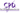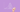Learn the basics of

# Probability & Statistical Analysis

The professional diploma in probability and statistical analysis module 1, will aim to give you a thorough understanding of all the fundamental concepts you need as an analyst. This module will include crucial theories, such as the Central Limit Theorem and Bayes Theorem, needed for those wanted to understand data in more depth. SAS Studio will cater to the practical understanding of the theoretical concepts studied throughout the module. This module is the building block for anyone who wishes to gain a more thorough understanding of the the behavior behind the trend.

612K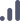All levels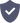Certified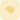# Course details

1.Introduction to Statistical Analysis

+

-

Lesson 1 will, in part, discuss what the difference between this course and the data analysis course is and who this course is aimed at. We will understand which fundamental concepts you need to lay for a good understanding of Statistical Analysis.

Thereafter, we will introduce the tool we will utilize throughout this module, called SAS Studio. We will end the lesson with a fun practical demonstration in SAS.

2.Understanding the Data

+

-

This lesson will be all about study design, data types and a sneak peak into summarizing data with the measures of central location. Understanding the study design and the type of scales that are used to measure data, is a crucial step in analysing the data accurately. Only after we know the pros and cons of the way the data was gathered, can we start describing the data.

3.Summarising Data

+

-

This lesson will mainly focus on the different methods of summarising data. The previous lesson introduced measures of central tendency to the student. Lesson 3 will elaborate on that concept with measures of spread as well as various ways of visualising data through plots in SAS Studio. Each topic will be consolidated through a practical demonstration in SAS Studio.

4.Probability Theory

+

-

This lesson will introduce the student to the concepts of probability theory. This lesson includes concepts like samples and populations. The lesson will define the basic definitions and rules of probability. This lesson will end by touching on more advanced concepts like mutually exclusive events, independent events, non independent events, and non mutually exclusive events.

5.Distributions

+

-

Lesson 5 aims to initiate the student's understanding of random variables and a various number probability distributions known and identified. Together with these concepts, this lesson will showcase the famous Central Limit Theorem (a fundamental concept to the understanding of sample sizes to be discussed in the next lesson).

6.Sample Sizes and Sampling

+

-

This lesson will answer the well known question of "how many observations does the study need in order to be statistically significant?". Many studies skip this fundamental step and end up not being able to prove statistical significance as a result. Lesson 6 will understand what it means for a result to be statistically significant and why it is so important for the sample to be large enough.

7.Hypothesis Testing

+

-

Lesson 7 will focus on questions about a single group. This lesson starts to uncover the concepts of inferential statistics; statistical methods used to draw conclusions from the sample in order to make conclusion about the population. Prior lessons focused on descriptive statistics, because they helped the student to describe and summarise the data through various methods like plots and summary statistics.

8.Hypothesis Testing Continued

+

-

This lesson will continue the concepts of hypothesis testing we introduced in lesson 7. We explore and investigate hypothesis testing concepts surrounding small and large samples. By the end of this lesson, you will be able to identify which procedure to use for a variety of different scenarios. Lesson 8 will end by tying together the practical concepts explored throughout Module 1 and offer some new tips and tricks for data management in SAS Studio not yet explored.

###### Certified by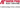###### Globally recognised by